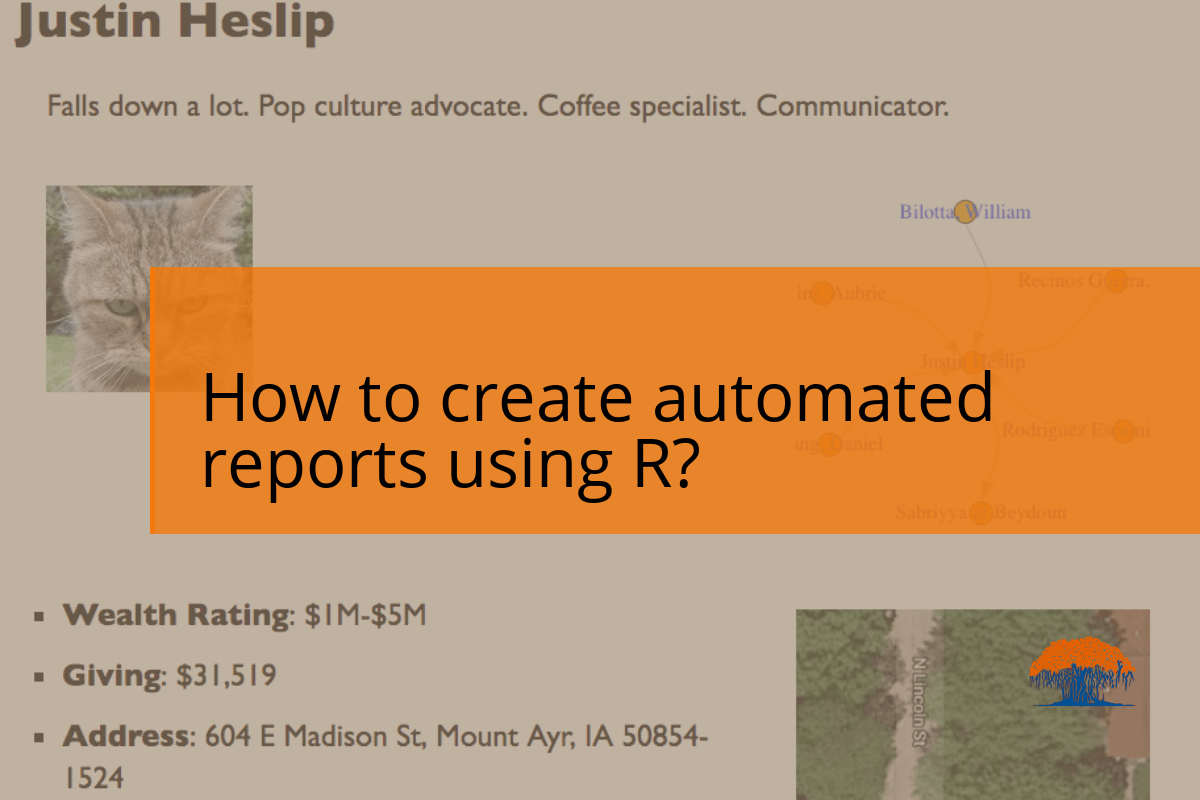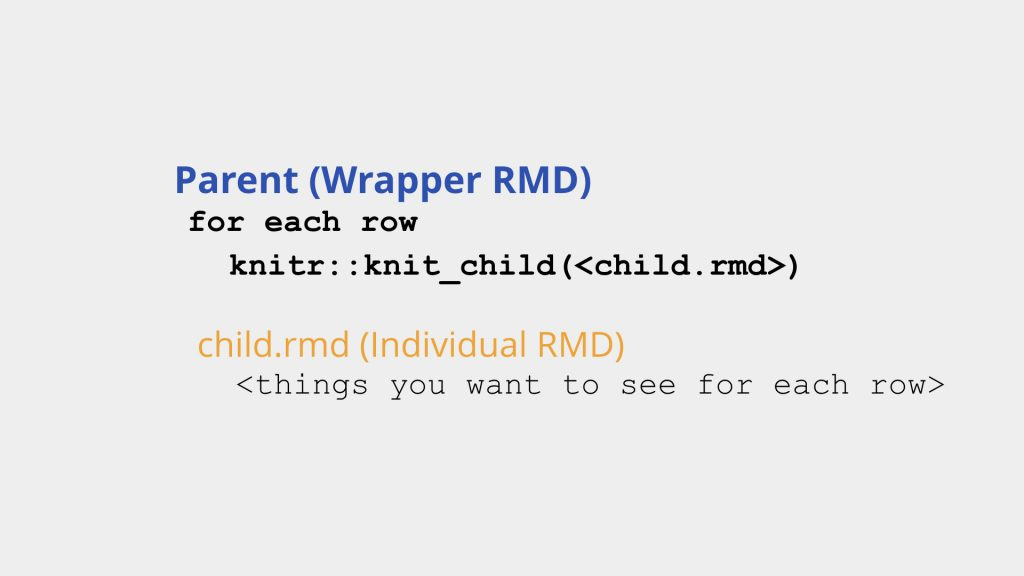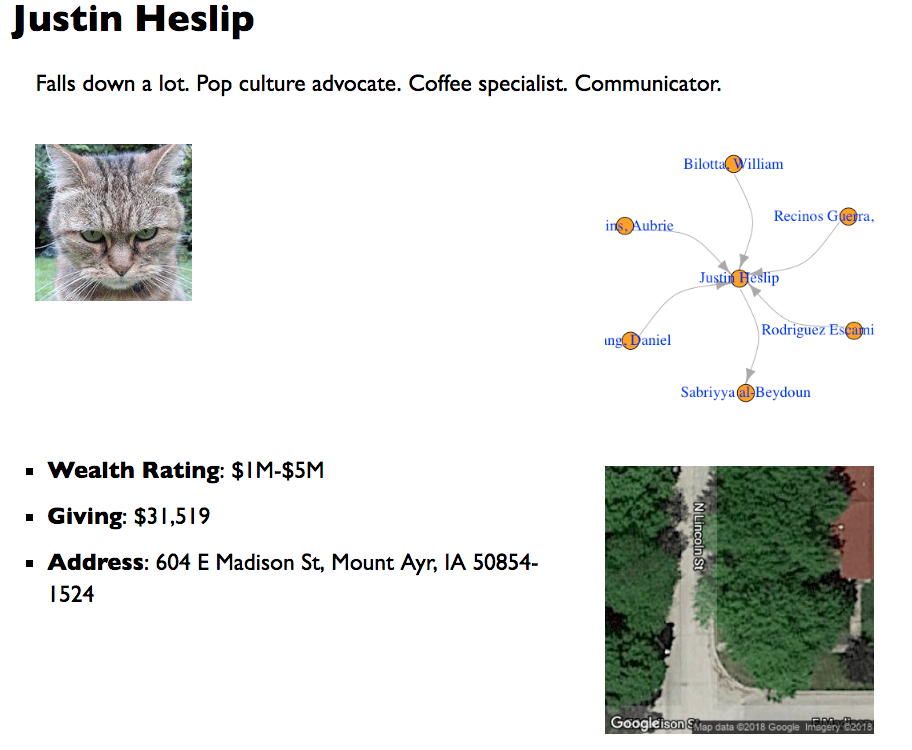Have you found yourself repeating the same analysis for different groups in your data? And have you wondered about a better way of doing so in R? I have this problem often. RMarkdown, knitr, and RStudio make generating such automated reports very easy.

You need two things:

• A parent file for data prep, loading libraries and knitting the final output
• A child file with the elements you want to see on the final reportLet’s see these files in detail. You can find these files here.

# Parent or Wrapper File

This file contains scripts to load and prep data, load relevant libraries, loop to go through each group of data, and knitting the final output.

Here’s what this file looks like for this example:

```--- title: "Briefings for an important event" output: slidy_presentation: keep_md: yes css: style.css ---     ```{r setup, include=FALSE} knitr::opts_chunk\$set(echo = FALSE, warning = FALSE, message = FALSE, cache = FALSE, fig.asp = 1)   #load the libraries library(tidyverse) library(ggmap) library(knitr) library(igraph) register_google(key = <your_key>, account_type = 'premium', day_limit = 100000)   # http://www.generatorland.com/glgenerator.aspx?id=124 # https://www.fakepersongenerator.com/user-biography-generator # https://fakena.me/fake-name/ # https://fakena.me/random-real-address/ # https://bit.ly/2INPTbT # https://www.nature.com/articles/sdata201575 # https://dataverse.harvard.edu/dataset.xhtml?persistentId=doi:10.7910/DVN/28201     # load the data bio_data <- readxl::read_excel('data/sample-data.xlsx')   network_data <- read_csv("data/sample_network.csv") vertices_data <- read_csv("data/sample_vertices.csv") ```   ```{r runall, include=FALSE} # run through each row of the dataset # https://stackoverflow.com/a/17105758 # https://stackoverflow.com/a/19156308 out <- NULL for (i in seq_len(nrow(bio_data))) { out <- c(out, knitr::knit_child('indiv-briefing.Rmd')) } ```   `r paste(out, collapse = '\n')````

# Child File

In this file, we specify each of the element we want to see in the report. In this example, we show photos, brief summaries, names, addresses, Google satellite view of the addresses, and a network graph. We named this file `indiv-briefing.Rmd`.

``````{r, echo=FALSE} data <- filter(bio_data, row_number() == i) ```   # `r as.character(select(data, name))` `r as.character(select(data, brief))`   <div class="dos-column-left"> ```{r, out.width="30%"} include_graphics("https://cataas.com/cat?type=sq") ``` </div>   <div class="dos-column-right"> ```{r, echo=FALSE, message=FALSE, fig.align='left', fig.width=3.5} par(mar = rep(0.1, 4)) network_data %>% filter(from == i | to == i) %>% graph_from_data_frame(d = ., vertices = filter(vertices_data, id %in% unlist(c(.\$from, .\$to), use.names = FALSE))) %>% plot.igraph(vertex.color = "orange", vertex.label.cex = 1.2, vertex.label.color = "blue", edge.curved = TRUE) ``` </div>   <div class="dos-column-left"> - **Wealth Rating**: `r as.character(select(data, rating))` - **Giving**: `r scales::dollar(as.numeric(select(data, giving)))` - **Address**: `r as.character(select(data, address))` </div>   <div class="dos-column-right"> ```{r, echo=FALSE, message=FALSE, fig.align='left', fig.width=3.5} ggmap(get_googlemap(as.character(select(data, address)), zoom = 20, maptype = "hybrid", size = c(300, 300), scale = 1), extent = "device") ``` </div>```

Now, if you knit the parent file, you should get a report that looks something like this:Here’s a video to walk through this process:

I hope this helps. What are some of the challenges you have faced while creating automated, repeatable analysis?